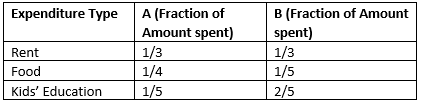# Rational numbers class 8 worksheet

In this page we have Rational numbers class 8 worksheet. We have given True and false questions, Multiple choice questions, Subjective questions based on properties of the rational numbers . Hope you like them and do not forget to like , social share and comment at the end of the page.

Question 1
Find the value of a
1. $\frac {1}{7} - \frac {5}{16 } = a$
2. $\frac {3}{13} - a =\frac { 20}{143}$
3. $a - \frac {1}{11 } = \frac {29}{165}$
4. $\frac {1}{3} - \frac {5}{13 } = a$
5. $\frac {5}{18} \times \frac {1}{4 } =a$
6. $\frac {5}{19} \times a =\frac { 5}{209}$
7. $a \times \frac {1}{5 } = \frac {1}{7}$

Question 2
Evaluate below expression
1. $\frac {1}{19} \times \frac {2}{9 }$
2. $\frac {2}{19} - \frac {1}{10 }$
3. $\frac {4}{13} \times \frac {1}{11 }$
4. $\frac { 3}{76} \div \frac {3}{8 }$
5. $\frac {2}{9} - \frac {5}{11 } =$
6. $\frac {4}{13} \times \frac {5}{16 } - \frac {5}{52}$
7. $\frac {1}{3} \times \frac {5}{11 } + \frac {5}{33}$
8. $\frac {1}{16} \times \frac {2}{19 } \div \frac { 1}{152}$
9. $\frac {1}{18} \times \frac {1}{5 } - \frac {1}{90}$
10. $\frac {3}{11} \times \frac {1}{9 }$
11. $\frac {1}{4} - \frac {1}{3 } - \frac {-1}{12}$
12. $\frac {4}{7} - \frac {4}{11 } + \frac {16}{77}$
13. $\frac {1}{19} - \frac {1}{15 } - \frac {-4}{285}$

Question 3
Find the additive inverse of each of the following:
1. $\frac {1}{2}$
2. $\frac {-2}{7}$
3. $\frac {-1}{11}$
4. 12
5. $\frac {-3}{10}$

Question 4
True or False Statement
1. Every rational number has a reciprocal
2. 3/2 lies between 1 and 2
3. The negative of 1 is 1 itself.
4. For all rational numbers a and b, a – b = b – a.
5. -1/2 is smaller than -2

Question 5
Find the multiplicative inverse of the following.
1. $\frac {-12}{11}$
2. $\frac {-1}{9}$
3. $\frac {2}{3}$
4. $\frac {-1}{2} \times \frac {-2}{5}$
5. $\frac {-1}{8} \times \frac {-8}{7}$
6. -11

## Multiple Choice Questions

Question 6
if a and b are positive numbers and a > b, then which of the is true?
(a) -a > -b
(b) 1/a > 1/b
(c) 1/b > 1/a
(d) -1/b > -1/a
Question 7
Which of the following is a rational number between 2/3 and 4/5?
(a) 1/2
(b) 1
(c) 2
(d) 41/60
Question 8
Multiplicative inverse of a negative rational number is
(a) a positive rational number.
(b) a negative rational number.
(c) 0
(d) 1
Question 9
which of the following is false?
(a) 9/6 lies between 1 and 2
(b) The multiplicative inverse of 3/4 is 4/3
(c) The reciprocal of 2/5 is 45/10
(d) None of the above
Question 10
The multiplicative inverse of $-1 \frac {2}{9}$ is
(a) -10/9
(b) -11/9
(c) -9/11
(d) 9/11
Question 11
The sum of the additive inverse and multiplicative inverse of 2 is
(a) 3/2
(b) -3/2
(d) 1/2
(d) 1
Question 12
The product of the additive inverse and multiplicative inverse of -1/2 is
(a) 1
(b) 2
(d) -1
(d) 0

## Subjective Type Questions

Question 13
Ram have a rope of 50 metres long and he cut the pieces of equal size from equal. If the length of the one of the piece is 5/3, how many pieces did he cut?

Question 14
Write the following rational numbers in the descending order.
8/7,-9/8 ,-3/2 ,0 , 1/2, 2/5

Question 15
A and B receives the same amount of Salary. Here are expenditures by each of them(i) If the amount left is gone to saving, what fraction of salary are A and B Saving?
(ii) If the Salary is Rs 60000, what is the saving in Rupees

## Summary

This Rational numbers class 8 worksheet is prepared keeping in mind the latest syllabus of CBSE . This has been designed in a way to improve the academic performance of the students. If you find mistakes , please do provide the feebback on the mail.